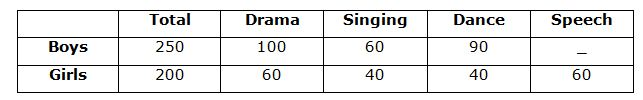# IBPS PO Prelims Quantitative Aptitude Questions 2019 (Day-8)

Dear Aspirants, Our IBPS Guide team is providing new series of Quantitative Aptitude Questions for IBPS PO Prelims 2019 so the aspirants can practice it on a daily basis. These questions are framed by our skilled experts after understanding your needs thoroughly. Aspirants can practice these new series questions daily to familiarize with the exact exam pattern and make your preparation effective.

Check here for IBPS PO Prelims Mock Test 2019

##### Check here for IBPS PO  Mock Test 2019
[WpProQuiz 7014]

Directions (1 – 5): What approximate value should come in the place of question mark in the given questions?

1) 3240 ÷ 30 * 15 =? * 16

a) 104

b) 101

c) 105

d) 106

d) 110

2) 61% of 985 – 151.046 =? – 63% of 698

a) 860

b) 880

c) 830

d) 850

e) 890

3) (447.68/32) ÷ (19.23/778.69) * (227.54/76) =?

a) 51

b) 54

c) 55

d) 58

e) 49

4) 1234.67 + 657.009 + 89.763 + 452.987 + 6753.099 =?

a) 9188

b) 9288

c) 8788

d) 8188

e) 9648

5) √7398 * √11025 + √627 =? – √731

a) 8082

b) 7982

c) 9082

d) 9882

e) 9152

Directions (6 – 10): Read the given following passage carefully and answer the given questions.

In the cultural function, 450 students participated. The ratio of the number of boys to the number of girls is 5: 4 respectively. Out of total girls 20% participated in singing and the remaining girls participated in dance, drama and speech in the ratio of 2: 3: 3. Two-five of the total boys participated in drama and the remaining boys participated in dance and singing in the ratio of 3: 2.

6) What is the total number of girls who have participated in Drama and Speech together?

a) 120

b) 100

c) 140

d) 160

e) None of these

7) What is the ratio of number of boys to the number of girls who have participated in Dance?

a) 3: 2

b) 9: 4

c) 5: 9

d) 4: 3

d) None of these

8) The average number of students participated in singing is what percent less than that of the average number of students participated in Drama?

a) 36.75%

b) 37.50%

c) 35.45%

d) 40.50%

d) None of these

9) The total number of students participated in speech is what percent of the total number of boys participated in the function?

a) 24

b) 25

c) 23

d) 27

e) None of these

10) What is the difference between the total number of students participated in Dance and Drama and the total number of students participated in Singing and Speech?

a) 140

b) 120

c) 150

d) 130

e) None of these

Directions (1-5):

? * 16 = (3240/30) * 15

? * 16 = 3240/2

? = 101(approx)

61% of 985 – 151.046 =? – 63% of 698

60/100 * 985 – 151 =? – 63/100 * 700

591 – 151 =? – 441

? = 881 = 880 (Approx.)

? = (448/32) * (779/19) * (228/76)

? = 14 + 41 + 3

? = 58

1234.67 + 657.009 + 89.763 + 452.987 + 6753.099 =?

1235 + 657 + 90 + 453 + 6753 =?

9188 =?

√7398 * √11025 + √627 =? – √731

√7396 * √11025 + √625 =? – √729

86 * 105 + 25 =? – 27

9030 + 25 + 27 =?

9082 =?

Directions (6 – 10):

Total students = 450

No of boys = 450/9 * 5 =250

No of girls = 450/9 * 4= 200

No of girls participated in Singing = 200*20/100 = 40

No of girls participated in dance = 160 * 2/8 = 40

No of girls participated in drama = 160 * 3/8 = 60

No of girls participated in speech = 160 * 3/8 = 60

No of boys participated in drama = 250 * 2/5 = 100

No of boys participated in singing = 150 * 2/5 = 60

No of boys participated in dance = 150 * 3/5 = 90Total number of girls participated in Drama and Speech = 60 + 60 = 120

The number boys participated in dance=90

The number girls participated in dance=40

Ratio = 90: 40 = 9: 4

The average number of students participated in singing = (60 + 40)/2 = 50

The average number of students participated in drama = (100 + 60)/2 = 80

Percentage = 100 * (80 – 50)/80 = 37.5%

The total number of students participated in speech = 60

The total number of boys = 250

Percentage = 100 * 60/250 = 24%

The total number of students participated in Dance and Drama

= (100 + 60 + 90 + 40) = 290

The total number of students participated in Singing and Speech

= (60 + 40 + 60) = 160

Difference = 290 – 160 = 130

5 1 vote
Rating